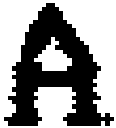# Fuzzy Skeletonization

The Fuzzy Skeletonization is an algorithm to get the skeleton of a bit image (black and white, greyscale or colored). This hybrid algorithm generates something like a distance value to the edges of the picture foreground. How to see in the 3D view, the skeleton is a subset of the ridges of the functional "mountain range" and "mountain peaks". Figure 3 shows the ridges for the directions (x, y, xy, -xy) and the peaks (bold squares). The skeleton can be found as the connections between the peaks: Just run in the direction of the rigde lines. The size of the square is calculated by the distance to the next edge.Original Picture 3D view analized view of fuzzy skeleton

This easy algorithm has the followin benefits:

• It is so simple, that it may be implemented in HW
• If not implemented in HW, it is possible to use parallel processor technology
• It is stable independed from input complexity
• The runtime is independed from input complexity
• Lines can be separeted by thickness